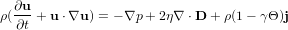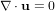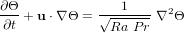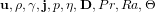## MIT Benchmark

#### Introduction

The MIT Benchmark 2001  describes heat driven cavity flow in a 8:1 rectangular domain at near-critical Rayleigh number. This was for special session entitled 'Computational Predictability of Natural Convection Flows in Enclosures' which was held at the First MIT Conference on Computational Fluid and Solid Mechanics. The primary objectives according to  are to identify the correct time-dependent solution for the 8:1 differentially-heated cavity with Ra=3.4×105 and Pr=0.71, to determine the critical Rayleigh number for the corresponding configuration, and to identify which methods can reliably provide these results. The Governing equations for this problem are the incompressible Navier-Stokes equation, continuity equation, and the energy equation written in terms of temperature$\begin{displaymath}\rho(\pp{\ub}{t}+\ub \cdot \nabla\ub) = -\nabla p + 2\eta \nabla \cdot\Db+ \rho (1-\gamma \Theta)\jb \end{displaymath}$$\begin{displaymath}\nabla\cdot\ub=0\end{displaymath}$$\begin{displaymath}\pp{\Theta}{t}+\ub \cdot \nabla\Theta = \frac{1}{\sqrt{Ra~Pr}}~\nabla^2\Theta \end{displaymath}$

where$\ub, \rho, \gamma, \jb, p, \eta, \Db, Pr, Ra, \Theta$ are the velocity, density, thermal expansion, gravity vector, pressure, viscosity, symmetric part of gradient velocity, Prandtl number, Rayleigh number and temperature (see  for details).

To solve the above set of PDEs, we employ a fully coupled monolithic approach utilizing Newton-multigrid solver with divided difference method in handling the jacobian. High order finite element space is implemented and second order time marching technique such as Crank-Nicolson is used. Both are meant to maintain the accuracy of the solution. Further details can be seen in .

» Continue to Geometry and Configuration